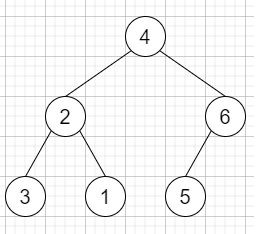# Construct Binary Tree from String in C++

Suppose we have a string consisting of parenthesis and integers. We have to construct a binary tree from that string. The whole input represents a binary tree. It holds an integer that followed by zero, one or two pairs of parentheses. The integer represents the root's value and a pair of parenthesis contains a child binary tree with the same structure.

So, if the input is like "4(2(3)(1))(6(5))", then the output will be [3,2,1,4,5,6] (inorder traversal)To solve this, we will follow these steps −

• Define a function solve(), this will take s, idx,

• if idx >= size of s, then −

• return null

• num := empty string

• while (idx < size of s and s[idx] is not equal to '(' and s[idx] is not equal to ')'), do −

• num := num + s[idx]

• (increase idx by 1)

• node = new node with value num

• if idx < size of s and s[idx] is same as '(', then −

• (increase idx by 1)

• left of node := solve(s, idx)

• (increase idx by 1)

• if idx < size of s and s[idx] is same as '(', then −

• (increase idx by 1)

• right of node := solve(s, idx)

• (increase idx by 1)

• return node

• From the main method do the following −

• idx := 0

• temp = new node with value -1

• return solve(s, idx)

## Example (C++)

Let us see the following implementation to get better understanding −

Live Demo

#include <bits/stdc++.h>
using namespace std;
class TreeNode{
public:
int val;
TreeNode *left, *right;
TreeNode(int data){
val = data;
left = NULL;
right = NULL;
}
};
void inord(TreeNode *root){
if(root != NULL){
inord(root->left);
cout << root->val << " ";
inord(root->right);
}
}
class Solution {
public:
TreeNode* solve(string s, int& idx){
if (idx >= s.size())
return NULL;
string num = "";
while (idx < s.size() && s[idx] != '(' && s[idx] != ')') {
num += s[idx];
idx++;
}
TreeNode* node = new TreeNode(stoi(num));
if (idx < s.size() && s[idx] == '(') {
idx++;
node->left = solve(s, idx);
idx++;
if (idx < s.size() && s[idx] == '(') {
idx++;
node->right = solve(s, idx);
idx++;
}
}
return node;
}
TreeNode* str2tree(string s) {
int idx = 0;
TreeNode* temp = new TreeNode(-1);
return solve(s, idx);
}
};
main(){
Solution ob;
TreeNode *root = ob.str2tree("4(2(3)(1))(6(5))");
inord(root);
}

## Input

"4(2(3)(1))(6(5))"

## Output

3 2 1 4 5 6